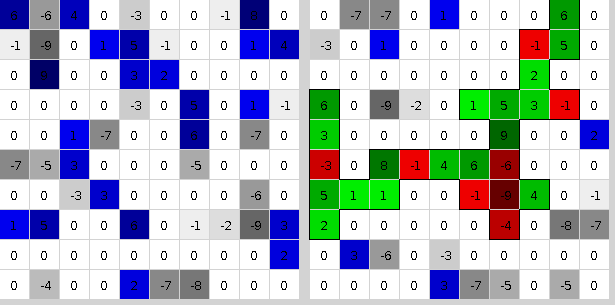# Marathon Match 94 - Marathon Match 94

## Key Information

Register
Submit
The challenge is finished.

## Challenge Overview

### Problem Statement

You are given an S x S matrix M. Each element of the matrix is an integer between -9 and 9, inclusive.

A permutation of numbers 0..S-1 p0, p1, ... pS-1 defines a new matrix MP as a permutation of rows and columns of the matrix M: the element of the new matrix in row i and column j MPi, j = Mp_i, p_j.

Consider all 4-connected regions of non-zero elements of the matrix MP which are bordered either by the boundaries of the matrix or by zero elements. Your task is to find a permutation which maximizes the sum of the elements multiplied by the square root of the number of elements in one of these connected regions. Note that you can't exclude negative elements at will; all non-zero elements of a region count towards the sum of its elements.

Here is an example for test 0 with p = (4 3 8 7 2 0 1 9 6 5).### Implementation

Your code should implement a single method permute(int[] m). m[i*S+j] = Mi, j (you can deduce S as sqrt(length(m))). You should return an array of S integers specifying the permutation p.

### Scoring

Your score for an individual test case will be the greatest of (the sum of the elements multiplied by square root of the number of elements) of the connected regions in the permuted matrix MP. If your return has invalid format or no connected regions in the matrix have positive sum of the elements, your score for the test case will be 0.

Your overall score will be calculated in the following way: for each test case where your score is not 0, you get 1 point for each competitor you beat on this test case (i.e., your score on a test case is larger than this competitor's score) and 0.5 points for each competitor you tie with (a tie with yourself is not counted); finally, the sum of points is divided by (the number of competitors - 1), then multiplied by 1000000 and divided by the number of test cases.

#### Tools

An offline tester is available here. You can use it to test/debug your solution locally. You can also check its source code for exact implementation of test case generation and score calculation. That page also contains links to useful information and sample solutions in several languages.

### Definition

 Class: ConnectedComponent Method: permute Parameters: int[] Returns: int[] Method signature: int[] permute(int[] m) (be sure your method is public)

### Notes

-The time limit is 10 seconds per test case (this includes only the time spent in your code). The memory limit is 1024 megabytes.
-There is no explicit code size limit. The implicit source code size limit is around 1 MB (it is not advisable to submit codes of size close to that or larger). Once your code is compiled, the binary size should not exceed 1 MB.
-The compilation time limit is 30 seconds. You can find information about compilers that we use and compilation options here.
-There are 10 example test cases and 100 full submission (provisional) test cases. There will be 2000 test cases in the final testing.
-The match is rated.

### Constraints

-S will be between 50 and 500, inclusive.

### Examples

0)

 `"1"`
```Returns:
"seed = 1
10
6 -6  4  0 -3  0  0 -1  8  0
-1 -9  0  1  5 -1  0  0  1  4
0  9  0  0  3  2  0  0  0  0
0  0  0  0 -3  0  5  0  1 -1
0  0  1 -7  0  0  6  0 -7  0
-7 -5  3  0  0  0 -5  0  0  0
0  0 -3  3  0  0  0  0 -6  0
1  5  0  0  6  0 -1 -2 -9  3
0  0  0  0  0  0  0  0  0  2
0 -4  0  0  2 -7 -8  0  0  0
"```
1)

 `"2"`
```Returns: "seed = 2
S = 50
"```
2)

 `"3"`
```Returns: "seed = 3
S = 500
"```
3)

 `"4"`
```Returns: "seed = 4
S = 102
"```
4)

 `"5"`
```Returns: "seed = 5
S = 120
"```
5)

 `"6"`
```Returns: "seed = 6
S = 411
"```
6)

 `"7"`
```Returns: "seed = 7
S = 218
"```
7)

 `"8"`
```Returns: "seed = 8
S = 354
"```
8)

 `"9"`
```Returns: "seed = 9
S = 357
"```
9)

 `"10"`
```Returns: "seed = 10
S = 270
"```

This problem statement is the exclusive and proprietary property of TopCoder, Inc. Any unauthorized use or reproduction of this information without the prior written consent of TopCoder, Inc. is strictly prohibited. (c)2020, TopCoder, Inc. All rights reserved.

## Payments

Topcoder will compensate members in accordance with our standard payment policies, unless otherwise specified in this challenge. For information on payment policies, setting up your profile to receive payments, and general payment questions, please refer to ‌Payment Policies and Instructions.

## LEARN:

Topcoder Challenges Explained